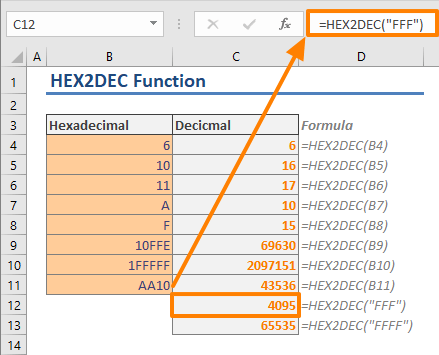The HEX2DEC is an Engineering function that converts a hexadecimal number to decimal. In this guide, we’re going to show you how to use the HEX2DEC function and also go over some tips and error handling methods.

## Supported versions

• All Excel versions

HEX2DEC(number)

## Arguments

 number The hexadecimal number you want to convert.

## HEX2DEC Function Example

### Basic Example

The HEX2DEC function is very easy to use. It takes a single argument only, as a hexadecimal number, and returns the given number’s decimal equivalent. On the other hand, do not let the name “hexadecimal number” mislead you. Because hexadecimal numbers can include letters between “A” to “F”, you should provide the hexadecimal number as a string. For example, “AA10” or “”1FFFFF”.### Fun usage

Although the function itself very straight forward in terms of how it works, you can combine this function with UNICHAR to generate emojis in your Excel worksheets. Inserting emojis in Excel is possible because each emoji is encoded as a Unicode character. The UNICHAR function can convert numeric values into the corresponding characters. However, the numeric code should be in decimal base while emojis are encoded in hexadecimal. Obviously, the HEX2DEC function can do this conversion.

=UNICHAR(HEX2DEC("1F644"))## Tips

• The number cannot contain more than 10 characters (40 bits). The most significant bit of the number is its sign. The remaining 39 bits are magnitude bits. Negative numbers are represented using two's-complement notation.
• You can convert a given decimal number into a hexadecimal with DEC2HEX
• Other related functions:

## Issues

### #NUM!

• If the number is not a valid hexadecimal number, HEX2DEC function returns the #NUM! error value.
• The number should be between 1FFFFFFF and FFE0000000. Otherwise, you will get #NUM! error.